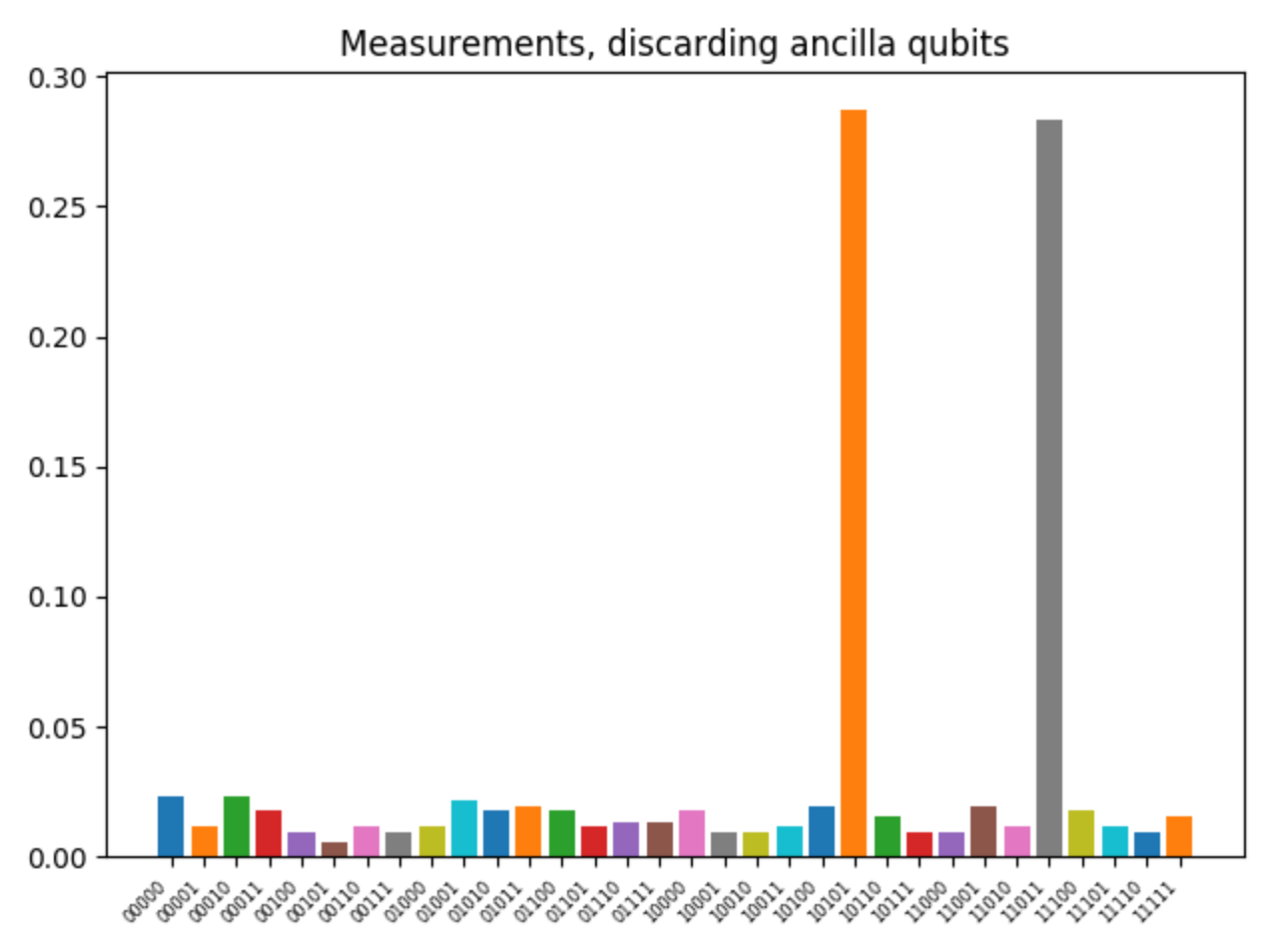# Code example: SAT

In this example we will utilize the SDK to solve a SAT problem on an emulator backend. It serves to show how you can use python to generate complicated qasm code. The example is written by David Bakker, Hitesh Dialani, Rembrandt Klazinga and Maurits van der Tol; four students following the Quantum Science and Quantum Information minor, TU Delft.

## Boolean satisfiability problem

The Boolean satisfiability problem, or SAT problem, is a classic problem in computer sciences. It is one of the problems where QPU's might be superior to their classical counterparts. The problem is simple: given a Boolean expression, is there a combination of Boolean variables for which the results is True? Is the expression satisfiable? For example, the expression

$\textit{f = a and b and not(c)}$

is satisfiable, because if we take

\begin{matrix} \begin{aligned} &\space\space\space a = \text{True}\ &\space\space\space b = \text{True}\ &\space\space\space c = \text{False}\ \end{aligned} && \text{than} && \begin{aligned} &f = \text{True} \end{aligned} \end{matrix}

The expression

$\textit{f = a and not(a)}$

is not satisfiable, as there is no value for a for which f = True. In this code example we will consider a specific version of the SAT problem. We will not determine whether or not the function is satisfiable, but we will look for the specific solution to a Boolean expression of which we assume it is satisfiable.

Try out the code in the docs of the quantuminspire repo to see the algorithm working. You should have successfully ran some of the simpler examples in the docs folder before attempting this one. See the README for an introduction.

The sat is solved using a Grover search algorithm. For each Boolean variable, a data qubit is initiated. Additionally, a final data qubit is initiated representing the outcome of the function f. The Boolean function is translated into logical gates working on the last data qubit. Depending on the function, multiple ancilla qubits might be necessary to encode the function. This encoding of the Boolean function is the oracle part of the Grover algorithm.

In the following example we run the algorithm 512 times, the solutions to the sat problem should be prominent among the results. We will evaluate the following Boolean function, to be defined in main.py:

        
boolean_expr = "(a and not(b) and c and d) or (not(a) and b and not(c) and d)"



The result of running main.py are displayed below:On the horizontal axis all the measured outcomes are listed. The first digit is the last data qubit and represents the function outcome f, hence it should be 1 for the outcome to be True. The most prominent peaks for which this is the case are solutions to the problem, in this case 1011 and 0101. These binaries represent the Boolean variables in alphabetical order. Respectively, the solutions are:

\begin{matrix} \begin{aligned} &\space\space\space a = \text{True}\ &\space\space\space b = \text{False}\ &\space\space\space c = \text{True}\ &\space\space\space d = \text{True}\ \end{aligned} \end{matrix}

and

\begin{matrix} \begin{aligned} &\space\space\space a = \text{False}\ &\space\space\space b = \text{True}\ &\space\space\space c = \text{False}\ &\space\space\space d = \text{True}\ \end{aligned} \end{matrix}

Upon checking, these solutions indeed satisfy the problem.

The reader is invited to explore the python functions used to generate the qasm code themselves, it may serve as a great inspiration to anybody looking to automate the generation of a qasm algorithm!

## A Boolean function as oracle

For those interested, lets shortly look at how the boolean function of the sat problem is implemented as oracle in the Grover algorithm. We use a slightly simpler function:

        
boolean_expr = "(a and not(b) and c)"



The qasm code generated looks like this:

The oracle part is the part of the algorithm in between the first and second column of H's, as in any Grover algorithm. The part after the second column can be recognised as the diffusion part of the algorithm. The Boolean function is split up in two parts. First 'not(b) and c' is evaluated and the result is stored in ancilla qubit q. Afterwards 'ancilla and a' is evaluated and stored in q, the 'result qubit', the result of the Boolean function. Because of Hadamards, the result is stored as a phase flip (multiply with -1) if and only if the results qubit was 1, otherwise there will be no effect. After each step in the algorithm, the step should be repeated in reversed order, making the full oracle symmetric. In this case there is only one step that should be reversed, as can be seen. This ensures that all qubits are set back to their original position, which is important for the diffusion part and the next cycles. So, for a state where the Boolean function results in True, and the result qubit is 1, the phase of the state will be flipped. In all other cases the state will keep it's original phase. This renders the exact same effect as an oracle, with as targets the solution of the sat problem.

As in any Grover’s algorithm, all possible states are 'tried out' simultaneously, by initiating with a column of Hadarmards, creating a completely homogeneous superstate of all possible states. The states that receive a phase flip by the oracle are subsequently emphasised by the diffusion step, and can thus afterwards be detected by a measurement.